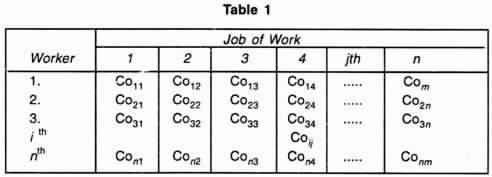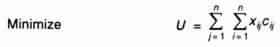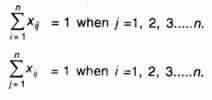# Assignment Problem: Hungarian Assignment Methods

Assignment problem is a special type of linear programming problem which deals with the allocation of the various resources to the various activities on one to one basis. It does it in such a way that the cost or time involved in the process is minimum and profit or sale is maximum. Though there problems can be solved by simplex method or by transportation method but assignment model gives a simpler approach for these problems.

In a factory, a supervisor may have six workers available and six jobs to fire. He will have to take decision regarding which job should be given to which worker. Problem forms one to one basis. This is an assignment problem.

1. ASSIGNMENT MODEL

Suppose there are n facilitates and n jobs it is clear that in this case, there will be n assignments. Each facility or say worker can perform each job, one at a time. But there should be certain procedure by which assignment should be made so that the profit is maximized or the cost or time is minimized.In the table, Coij is defined as the cost when jth job is assigned to ith worker. It maybe noted here that this is a special case of transportation problem when the number of rows is equal to number of columns.

Mathematical Formulation

Any basic feasible solution of an Assignment problem consists (2n – 1) variables of which the (n – 1) variables are zero, n is number of jobs or number of facilities. Due to this high degeneracy, if we solve the problem by usual transportation method, it will be a complex and time consuming work. Thus a separate technique is derived for it. Before going to the absolute method it is very important to formulate the problem.

Suppose xjj is a variable which is defined as

1 if the ith job is assigned to jth machine or facility

0 if the ith job is not assigned to jth machine or facility.

Now as the problem forms one to one basis or one job is to be assigned to one facility or machine.The total assignment cost will be given byThe above definition can be developed into mathematical model as follows:

Determine xij > 0 (i, j = 1,2, 3…n) in order toSubjected to constraintsand xij is either zero or one.

### Method to solve Problem (Hungarian Technique)

Consider the objective function of minimization type. Following steps are involved in solving this Assignment problem,

1. Locate the smallest cost element in each row of the given cost table starting with the first row. Now, this smallest element is subtracted form each element of that row. So, we will be getting at least one zero in each row of this new table.
2. Having constructed the table (as by step-1) take the columns of the table. Starting from first column locate the smallest cost element in each column. Now subtract this smallest element from each element of that column. Having performed the step 1 and step 2, we will be getting at least one zero in each column in the reduced cost table.
3. Now, the assignments are made for the reduced table in following manner.

(i) Rows are examined successively, until the row with exactly single (one) zero is found. Assignment is made to this single zero by putting square around it and in the corresponding column, all other zeros are crossed out (x) because these will not be used to make any other assignment in this column. Step is conducted for each row.

(ii) Step 3 (i) in now performed on the columns as follow:- columns are examined successively till a column with exactly one zero is found. Now , assignment is made to this single zero by putting the square around it and at the same time, all other zeros in the corresponding rows are crossed out (x) step is conducted for each column.

(iii) Step 3, (i) and 3 (ii) are repeated till all the zeros are either marked or crossed out. Now, if the number of marked zeros or the assignments made are equal to number of rows or columns, optimum solution has been achieved. There will be exactly single assignment in each or columns without any assignment. In this case, we will go to step 4.

1. At this stage, draw the minimum number of lines (horizontal and vertical) necessary to cover all zeros in the matrix obtained in step 3, Following procedure is adopted:

(i) Tick mark () all rows that do not have any assignment.

(ii) Now tick mark() all these columns that have zero in the tick marked rows.

(iii) Now tick mark all the rows that are not already marked and that have assignment in the marked columns.

(iv) All the steps i.e. (4(i), 4(ii), 4(iii) are repeated until no more rows or columns can be marked.

(v) Now draw straight lines which pass through all the un marked rows and marked columns. It can also be noticed that in an n x n matrix, always less than ‘n’ lines will cover all the zeros if there is no solution among them.

1. In step 4, if the number of lines drawn are equal to n or the number of rows, then it is the optimum solution if not, then go to step 6.
2. Select the smallest element among all the uncovered elements. Now, this element is subtracted from all the uncovered elements and added to the element which lies at the intersection of two lines. This is the matrix for fresh assignments.
3. Repeat the procedure from step (3) until the number of assignments becomes equal to the number of rows or number of columns.

## One thought on “Assignment Problem: Hungarian Assignment Methods”

error: Content is protected !!